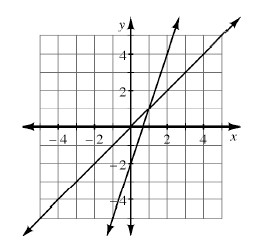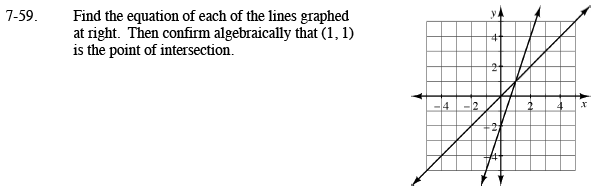Home > CAAC > Chapter 7 > Lesson 7.2.1 > Problem7-59

7-59.

Find the equation of each of the lines graphed below. Then confirm algebraically that (1, 1) is the point of intersection. Homework Help ✎Use y = mx + b.
You need to find the slope and y-intercept of each line.

For one of the lines, the slope is 3 and the y-intercept is −2.
Therefore its equation in y = 3x − 2. Now write the equation of the other line.

Use the Equal Values Method to confirm that (1, 1) is the point of intersection.